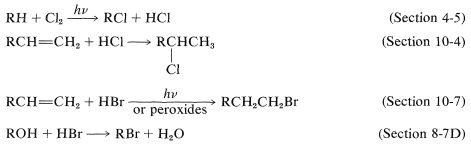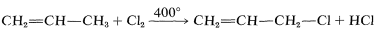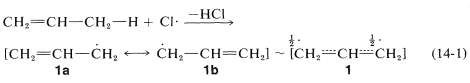# 14.4: Alkyl Halides

$$\newcommand{\vecs}{\overset { \rightharpoonup} {\mathbf{#1}} }$$ $$\newcommand{\vecd}{\overset{-\!-\!\rightharpoonup}{\vphantom{a}\smash {#1}}}$$$$\newcommand{\id}{\mathrm{id}}$$ $$\newcommand{\Span}{\mathrm{span}}$$ $$\newcommand{\kernel}{\mathrm{null}\,}$$ $$\newcommand{\range}{\mathrm{range}\,}$$ $$\newcommand{\RealPart}{\mathrm{Re}}$$ $$\newcommand{\ImaginaryPart}{\mathrm{Im}}$$ $$\newcommand{\Argument}{\mathrm{Arg}}$$ $$\newcommand{\norm}{\| #1 \|}$$ $$\newcommand{\inner}{\langle #1, #2 \rangle}$$ $$\newcommand{\Span}{\mathrm{span}}$$ $$\newcommand{\id}{\mathrm{id}}$$ $$\newcommand{\Span}{\mathrm{span}}$$ $$\newcommand{\kernel}{\mathrm{null}\,}$$ $$\newcommand{\range}{\mathrm{range}\,}$$ $$\newcommand{\RealPart}{\mathrm{Re}}$$ $$\newcommand{\ImaginaryPart}{\mathrm{Im}}$$ $$\newcommand{\Argument}{\mathrm{Arg}}$$ $$\newcommand{\norm}{\| #1 \|}$$ $$\newcommand{\inner}{\langle #1, #2 \rangle}$$ $$\newcommand{\Span}{\mathrm{span}}$$$$\newcommand{\AA}{\unicode[.8,0]{x212B}}$$

The important chemistry of alkyl halides, $$\ce{RX}$$, includes the nucleophilic $$\left( S_\text{N} \right)$$ displacement and elimination $$\left( E \right)$$ reactions discussed in Chapter 8. Recall that tertiary alkyl halides normally are reactive in ionization $$\left( S_\text{N}1 \right)$$ reactions, whereas primary halides, and to a lesser extent secondary halides, are reactive in $$S_\text{N}2$$ reactions, which occur by a concerted mechanism with inversion of configuration (Sections 8-4 to 8-7).

Elimination competes with substitution in many $$S_\text{N}$$ reactions and can become the major pathway at high temperatures or in the presence of strong base. Elimination $$\left( E_2 \right)$$, unlike displacement $$\left( S_\text{N}2 \right)$$, is insensitive to steric hindrance in the alkyl halide. In fact, the $$E_2$$ reactivity of alkyl halides is tert $$\ce{RX} >$$ sec $$\ce{RX} >$$ prim $$\ce{RX}$$, which is opposite to their $$S_\text{N}2$$ reactivity.Figure 14-1: Infrared and proton nmr spectra of substance $$\ce{C_4H_7Br}$$ and substance $$\ce{C_5H_8Br_2}$$. NMR spectra are at $$60 \: \text{MHz}$$ with reference to TMS at 0.0.

Several useful reactions for the synthesis of alkyl halides that we already have encountered are summarized below with references to the sections that supply more detail:A summary of these and some other reactions for the synthesis of alkyl halides or organohalogen compounds is given in Table 14-5.

## Allylic (2-Propenyl) Halides

Halogen compounds in which the carbon-halogen bond is adjacent to a double bon, as inare known as allylic halides. The simplest example is 3-chloropropene, $$\ce{CH_2=CHCH_2Cl}$$, which is made on a large scale by the radical chlorination of propene at $$400^\text{o}$$:Most of the 3-chloropropene prepared in this manner is converted to other important compounds. For example, addition of hypochlorous acid gives a mixture of dichloropropanols, which on treatment with base gives a substance known commercially as "epichlorohydrin":The ring closure reaction with $$\ce{Ca(OH)_2}$$ is an internal $$S_\text{N}2$$ reaction. Hydroxide ion converts the alcohol to an alkoxide ion that acts as a nucleophile in displacing the neighboring chlorine:The epichlorohydrice so produced is used primarily to make epoxy resins (see Section 29-5E), although some of it is hydrolyzed to glycerol:A general method for preparing allylic halides is by addition of hydrogen halides to conjugated dienes. This reaction usually produces a mixture of 1,2- and 1,4-addition products (see Section 13-2):A second general method involves the bromination of alkene with N-bromosuccinimide (the Wohl-Ziegler reaction). A radical-chain reaction takes place between N-bromosuccinimide (NBS) and alkenes, which commonly is initiated by light, peroxides, or other catalysts, and yields allylic bromides:This reaction, like the chlorination of propene, is highly selective in that the so-called allylic $$\ce{C-H}$$ is attacked preferentially.

From bond energies (Table 4-6) we know that the weakest $$\ce{C-H}$$ bonds of propene are to the allylic hydrogens, $$\ce{H_2C=CHCH_2-H}$$. Therefore, in the first step of radical-chain chlorination of propene, an allylic hydrogen is removed by a chlorine atom (Equation 14-1). The allylic $$\ce{C-H}$$ bonds are weaker than the alkenic $$\ce{C-H}$$ bonds because of the extra stabilization of the radical obtained on hydrogen abstraction (Equation 14-1). Two equivalent valence-bond structures ($$1a$$ and $$1b$$) can be written for the 2-propenyl radical; the electron delocalization enhances the stability of the radical (see Section 6-5C):In the second step of the chain reaction (Equation 14-2) the propenyl radical can form a carbon-halogen bond at either end by abstracting a halogen atom from the halogenating agent:The $$\ce{Cl} \cdot$$ atom produced now can participate in Reaction 14-1, thereby continuing the chain. With propene the intermediate radical gives the same product, 2-propenyl chloride, irrespective of whether a chlorine atom is transferred to the 1- or 3-carbon. However, the radical formed by removal of an allylic hydrogen from 2-butene gives a mixture of products:## $$S_\text{N}$$ Reactions of Allylic Halides

The carbon-halogen bonds of allylic halides are especially reactive in both $$S_\text{N}1$$ and $$S_\text{N}2$$ reactions (Table 14-6). The reasons for the enhanced $$S_\text{N}1$$ reactivity have been discussed previously (Section 8-7B). For example, the ease with which 1-chloro-2-butene ionizes compared to 1-chlorobutane is attributed to the stability of the 2-butenyl cation, which is distributed between $$\ce{C_1}$$ and $$\ce{C_3}$$, and the nucleophile (water) attacks at both positions to give mixtures of products. The same results are obtained if one starts with 3-chloro-1-butene because the same cation is formed:Figure 14-2: Atomic-orbital representation of the transition state for $$S_\text{N}2$$ displacement of 3-chloropropene (allyl chloride) with iodide ion. The halide orbitals are represented as spherical for simplicity. The purpose of this figure is to show the $$\pi$$ bonding, which can stabilize the transition state.

## Benzylic (Phenylmethyl) Halides

Reactivities comparable to allylic halides are found in the nucleophile displacement reactions of benzylic halides by $$S_\text{N}1$$ and $$S_\text{N}2$$ mechanisms (Table 14-6). The ability of the benzylic halides to undergo $$S_\text{N}1$$ reactions clearly is related to the stability of the resulting benzylic cations, the electrons of which are extensively delocalized. Thus, for phenylmethyl chloride,When the halogen substituent is located two or more carbons from the aryl group as in 2-phenylethyl bromide, $$\ce{C_6H_5CH_2CH_2Br}$$, the pronounced activating effect evident in benzylic halides disappears, and the reactivity of the halides is essentially that of a primary alkyl halide (e.g., $$\ce{CH_3CH_2CH_2Br}$$).

Benzylic halides can be prepared by the same radical-halogenating agents that give allylic halides from alkenes. These include $$\ce{Cl_2}$$, $$\ce{Br_2}$$, N-bromosuccinimide (Section 14-3A), $$\ce{SO_2Cl_2}$$, and tert-butyl hypochlorite:The benzylic $$\ce{C-H}$$ bond is weaker and more restrictive then primary alkane $$\ce{C-H}$$ bonds because of the stabilization of benzylic radicals (see Table 4-6).